PSA9CDE - Student-Facing Tasks---7.1 Lesson 5: The Size of the Scale Factor: Student Facing Tasks (7.G.A.1)
Part A)

Solve each equation mentally.

16176

Type your answer below as a number (example: 5, 3.1, 4 1/2, or 3/2):
Part B)

168

Type your answer below as a number (example: 5, 3.1, 4 1/2, or 3/2):
Part C)

161

Type your answer below as a number (example: 5, 3.1, 4 1/2, or 3/2):
Part D)Type your answer below as a number (example: 5, 3.1, 4 1/2, or 3/2):
Part E)Type your answer below as a number (example: 5, 3.1, 4 1/2, or 3/2):
Part A)

Your teacher will give you a set of cards. On each card, Figure A is the original and Figure B is a scaled copy.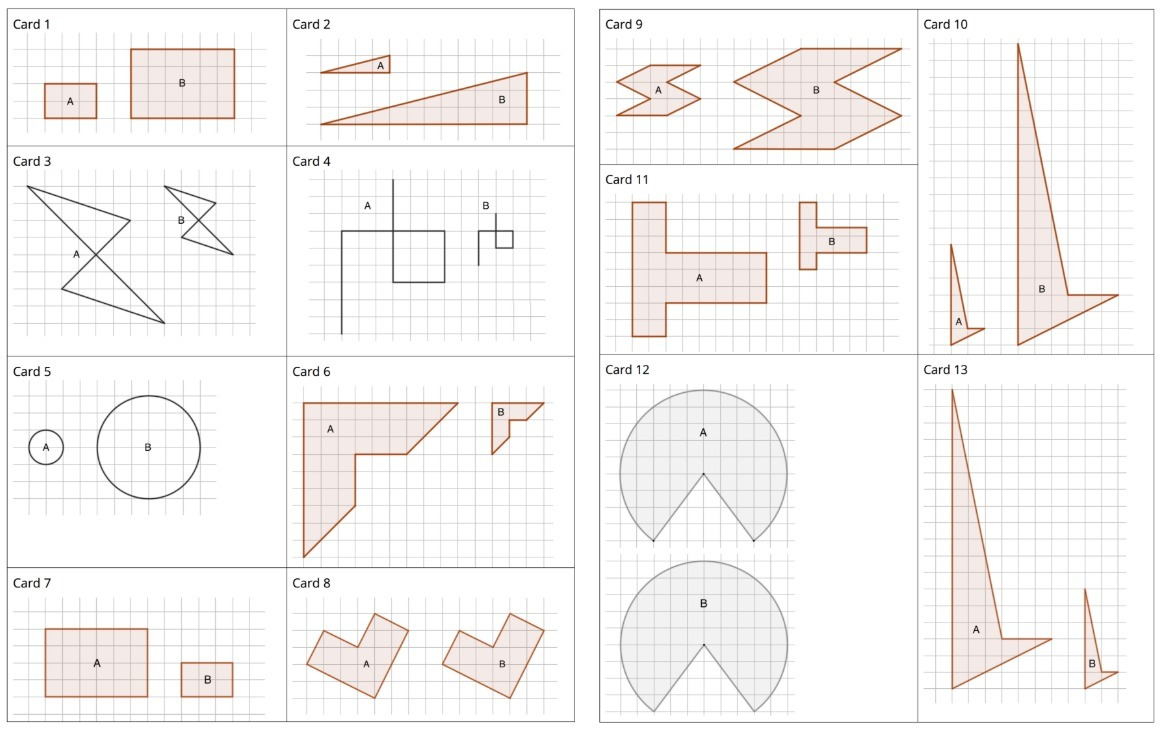Sort the cards based on their scale factors. Be prepared to explain your reasoning.

Part B)

Examine cards 10 and 13 more closely. What do you notice about the shapes and sizes of the figures? What do you notice about the scale factors?

Part C)

Examine cards 8 and 12 more closely. What do you notice about the figures? What do you notice about the scale factors?

Part A)

Your teacher will give you 2 pieces of a 6-piece puzzle.

If you drew scaled copies of your puzzle pieces using a scale factor of 1/2, would they be larger or smaller than the original pieces?

Select one:
Part B)

How do you know?

Part C)

Create a scaled copy of each puzzle piece on a blank square, with a scale factor of.

Draw your scaled copy on paper, take a picture, and upload it using the image upload icon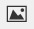If you do not have the ability to upload an image of your work type "Scaled copy is on paper."

Part D)

When everyone in your group is finished, put all 6 of the original puzzle pieces together like this:Next, put all 6 of your scaled copies together. Compare your scaled puzzle with the original puzzle. Which parts seem to be scaled correctly and which seem off? What might have caused those parts to be off?

Revise any of the scaled copies that may have been drawn incorrectly.

Part E)

If you were to lose one of the pieces of the original puzzle, but still had the scaled copy, how could you recreate the lost piece?

Part A)

What is the scale factor from the original triangle to its copy?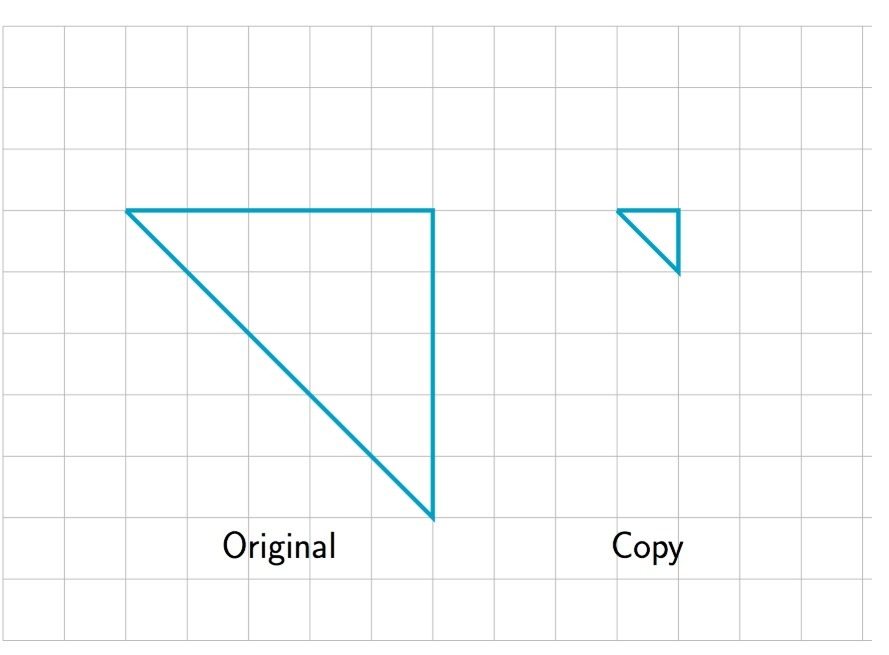Type your answer below as a number (example: 5, 3.1, 4 1/2, or 3/2):
Part B)

The scale factor from the original trapezoid to its copy is 2. Draw the scaled copy.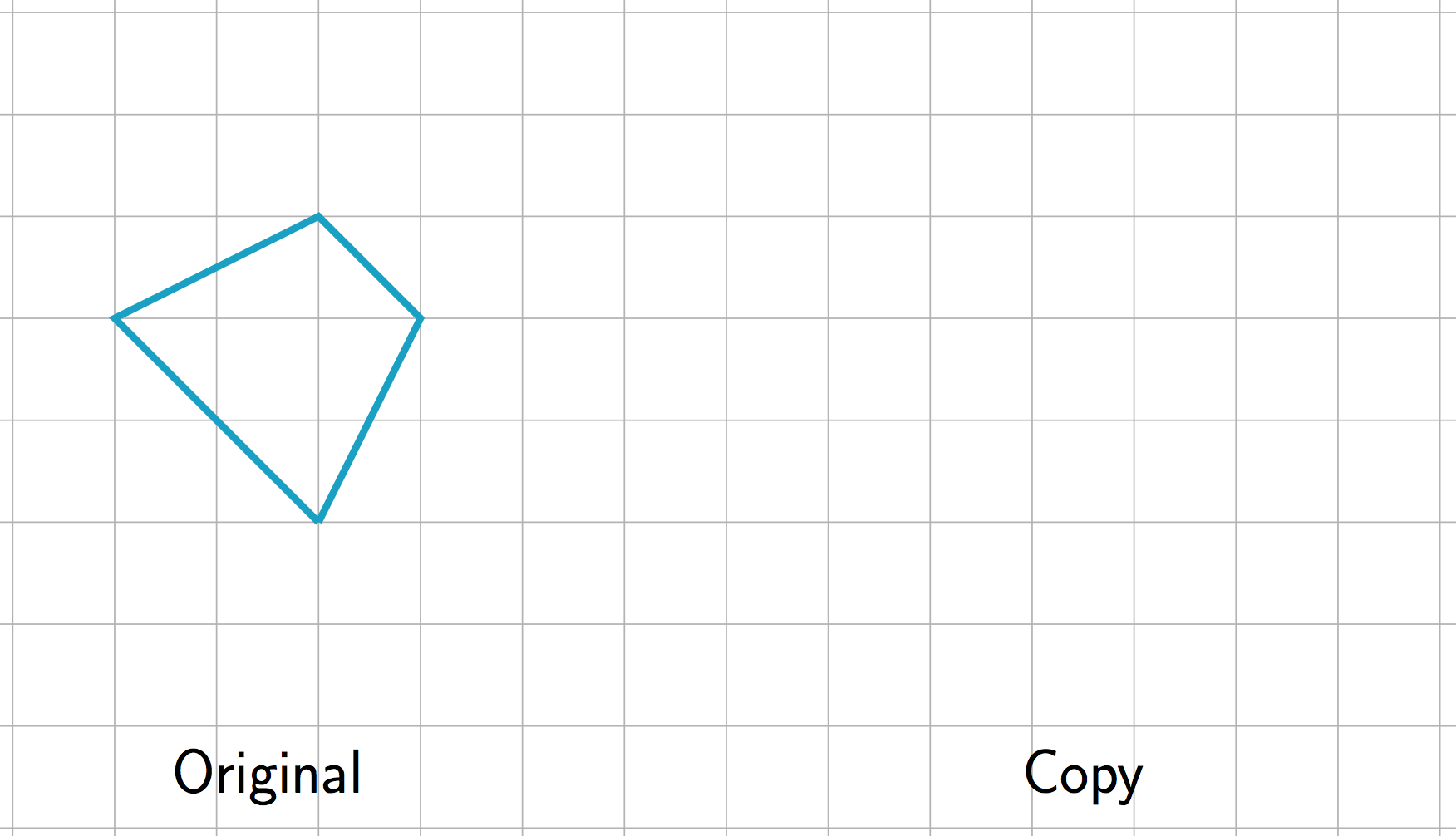Draw your scaled copy on paper, take a picture, and upload it using the image upload iconIf you do not have the ability to upload an image of your work type "Scaled copy is on paper."

Part C)

The scale factor from the original figure to its copy is. Draw the original figure.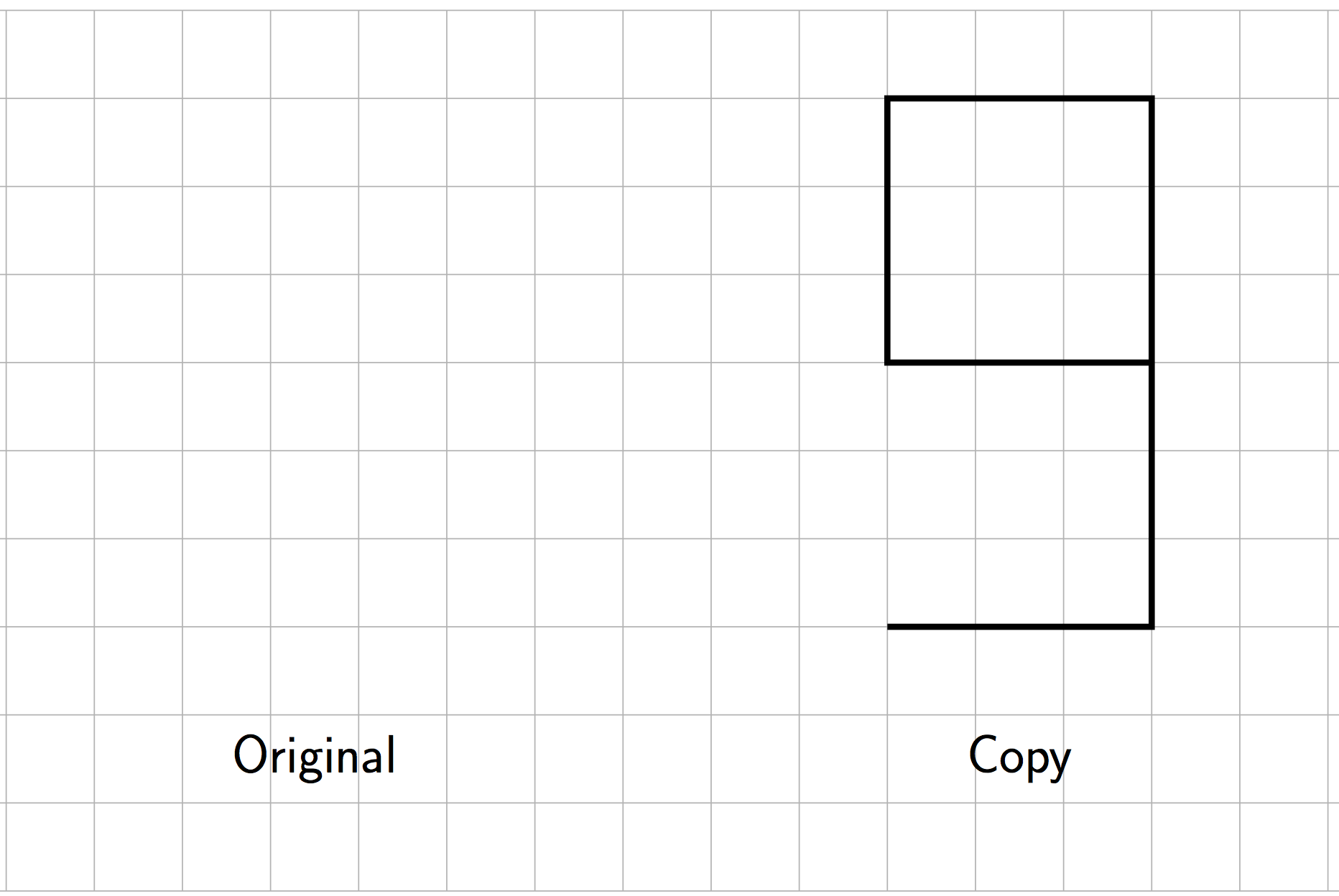Draw your original figure on paper, take a picture, and upload it using the image upload iconIf you do not have the ability to upload an image of your work type "Figure is on paper."

Part D)

What is the scale factor from the original figure to the copy?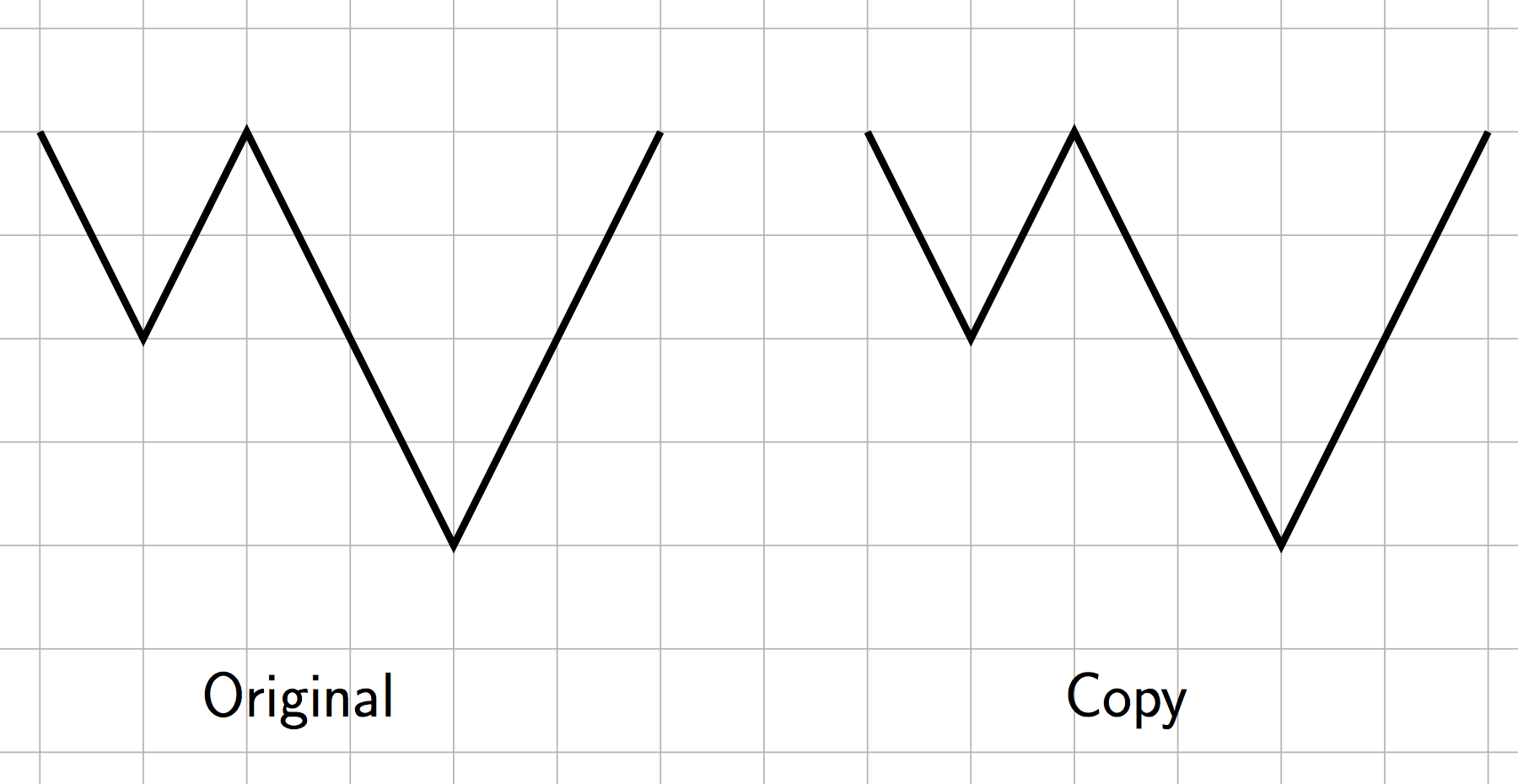Type your answer below as a number (example: 5, 3.1, 4 1/2, or 3/2):
Part E)

Explain how you know.

Part F)

The scale factor from the original figure to its scaled copy is 3. Draw the scaled copy.Draw your scaled copy on paper, take a picture, and upload it using the image upload iconIf you do not have the ability to upload an image of your work type "Scaled copy is on paper."

Part A)

A rectangle that is 2 inches by 3 inches has been scaled by a factor of 7.

What are the side lengths of the scaled copy?

Give the smaller length. Do not include units (inches) in your answer.